# gauge theory

(redirected from Yang-Mills action)
Also found in: Dictionary.
Related to Yang-Mills action: Gauge potential

## Gauge theory

The theoretical foundation of the four fundamental forces of nature, the electromagnetic, weak, strong, and gravitational interactions. Gauge symmetry lies at the heart of gauge theory. A gauge symmetry differs from an ordinary symmetry in two important respects:

1. Gauge symmetry is a local symmetry rather than a global symmetry. For a local symmetry, the element of the symmetry group (G) that acts on the fields of a theory at a space-time point ( x , t) depends on the position x and time t, whereas for a global symmetry a fixed group element acts on fields at different space-time points.

2. A gauge transformation leaves a physical state invariant. Gauge symmetry reflects a redundancy in the variables used to describe a physical state. By contrast, a global symmetry acting on a physical state in general produces a new, distinct physical state. See Fundamental interactions, Space-time

The simplest example of a gauge theory is electromagnetism. In classical electrodynamics, gauge invariance reflects the arbitrariness that exists in choosing the potentials A ( x , t) and &phgr;( x , t) to represent the electric and magnetic fields, E and B , according to Eqs. (1), where c is the speed of light. If &Lgr;( x , t) is an arbitrary scalar field,

(1)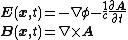(2)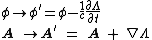Eqs. (2) define a gauge transformation. The potentials A and &phgr; may equally well be used to represent the electromagnetic fields E and B . See Maxwell's equations, Potentials, Relativistic electrodynamics

In nonrelativistic quantum mechanics, gauge invariance is realized as follows. The Schrödinger equation for a particle with an electromagnetic charge q and mass m is Eq. (3),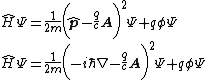(3)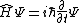where is the hamiltonian operator, is the momentum operator, &PSgr; is the wave function of the particle, and &planck; is Planck's constant divided by 2&pgr;. Equation (3) is invariant under the gauge transformation given by Eqs. (4).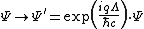(4)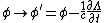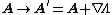Electromagnetism is a U(1) gauge symmetry [where U(1) is the one-dimensional unitary group, which is represented by the complex numbers ei&phiv; with 0 ≤ &phiv; < 2&pgr;] because a gauge transformation rotates the phase of the wave function in a space-time–dependent manner and adjusts the potentials A and &phgr; accordingly. See Quantum mechanics, Schr&ouml;dinger's wave equation, Unitary symmetry

The concept of a gauge theory may be generalized to larger, nonabelian Lie groups, such as G = SU(2), SU(3), SU(5), or SO(10). In 1954 C. N. Yang and R. L. Mills suggested gauging the SU(2) isospin symmetry, thus developing the first nonabelian gauge theory, also known as Yang-Mills theory. In 1971 G. `t Hooft demonstrated the renormalizability of nonabelian gauge theory. Nonabelian gauge theory is the foundation of the electroweak and strong interactions. In the electroweak theory, formulated by S. Weinberg and A. Salam in 1967, the gauge group G = SU(2)L × U(1)Y is spontaneously broken to U(1)Q, the gauge group of ordinary electromagnetism, by the condensation of a Higgs field. There are four kinds of particles that mediate the gauge interactions, called gauge bosons: two massive charged weak gauge bosons, the W+ and W-, with a mass of 80.4 GeV; a neutral gauge boson, the Z, with a mass of 91.2 GeV; and finally a massless photon (γ). The W± and the Z are responsible for the charged and neutral weak interactions, respectively, and the photon is the gauge particle responsible for the electromagnetic interaction. In quantum chromodynamics (QCD) the symmetry group is SU(3)color, which remains unbroken. There are eight gauge bosons called gluons. Quarks, which come in three colors, carry a color charge, and the quark field forms an SU(3) triplet. See Electroweak interaction, Symmetry breaking

One remarkable property of quantum chromodynamics is that color charge is antiscreened rather than screened, a feature arising from the nonabelian character of the gauge symmetry. This situation is opposite to that in QED. Consequently, as shorter distance scales are probed, the coupling constant αQCD decreases, so that at very short distances QCD approaches a free (noninteracting) field theory. This feature, pointed out in 1973 by D. Gross and F. Wilczek and by H. D. Politzer, is known as asymptotic freedom. Although on large scales QCD is a strongly coupled theory, at very small scales, which may be probed by scattering at very high energies, the constituents of hadrons (quarks and gluons) behave almost as if they were free particles, originally called partons. Similarly, at larger distances scales αQCD becomes larger, and perturbation theory breaks down. This increase in the coupling constant at large distances leads to a phenomenon known as confinement, which prevents colored objects (such as quarks or gluons) from being isolated. See Gluons, Quantum chromodynamics, Quarks

McGraw-Hill Concise Encyclopedia of Physics. © 2002 by The McGraw-Hill Companies, Inc.

## gauge theory

[′gāj ‚thē·ə·rē]
(physics)
Any field theory in which, as the result of the conservation of some quantity, it is possible to perform a transformation in which the phase of the fields is altered by a function of space and time without altering any measurable physical quantity, so that the fields obtained by any such transformation give a valid description of a given physical situation.
McGraw-Hill Dictionary of Scientific & Technical Terms, 6E, Copyright © 2003 by The McGraw-Hill Companies, Inc.
Site: Follow: Share:
Open / Close